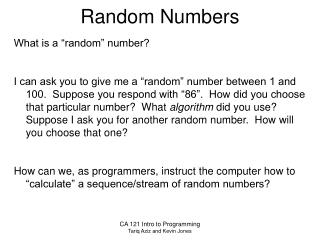DownloadDownload PresentationRandom Numbers

# Random Numbers

Télécharger la présentation## Random Numbers

- - - - - - - - - - - - - - - - - - - - - - - - - - - E N D - - - - - - - - - - - - - - - - - - - - - - - - - - -
##### Presentation Transcript

1. Random Numbers What is a “random” number? I can ask you to give me a “random” number between 1 and 100. Suppose you respond with “86”. How did you choose that particular number? What algorithm did you use? Suppose I ask you for another random number. How will you choose that one? How can we, as programmers, instruct the computer how to “calculate” a sequence/stream of random numbers? CA 121 Intro to Programming Tariq Aziz and Kevin Jones

2. Random Numbers Sometimes we, as programmers, need to generate random numbers. For example, in the Birthday Problem, we need to generate a “random” birthday for each person. We can do this simply by generating a “random” number between 1 and 365, inclusive. We can ask the computer to give us a “random” number between 0.0 and 1.0, [0.0, 1.0), and then we can scale the number to get it into a range that we require. In Visual Basic, the function Rnd() will return a number between [0.0, 1.0). CA 121 Intro to Programming Tariq Aziz and Kevin Jones

3. Random Numbers • So, if we want a random integer between 1 and 365, we can do the following: • Dim birthday As Integer • birthday = int( Rnd() * 365 ) + 1 • Let’s look at the right-hand side above in detail: • Rnd() gives us a “random” number between [0.0, 1.0) • Multiplying by 365 gives us a number between [0.0, 365.0) • Conversion to int gives us an int between [0, 364] • Finally, adding 1 gives us an int between [1, 365]. CA 121 Intro to Programming Tariq Aziz and Kevin Jones

4. MidSquare Method There are many ways to generate “random” numbers. We’ll take a closer look at the Midsquare method of generating random numbers between [0.0, 1.0). First, we start with a four-digit seed value, for example, 7182. We then square it to get a number up to eight digits long. If the number has fewer than eight digits, we pad the left with zeroes until we get eight digits. In our example, 7182^2 gives us 51581124. Now we choose the “middle” four digits of our result, which is 5811 in our example. We divide by 10,000 to get our first random number, e.g. 0.5811. We repeat this process indefinitely… CA 121 Intro to Programming Tariq Aziz and Kevin Jones

5. MidSquare Method CA 121 Intro to Programming Tariq Aziz and Kevin Jones

6. Pseudo-random Numbers It is important that the stream of pseudo-random numbers that we generate appear random, i.e. there is no discernable pattern to the numbers. Consider the stream generated by our previous example: 0.5811, 0.7677, 0.9363, 0.6657, 0.3156, 0.9603, 0.2176, 0.7349, … Can you find any pattern in the numbers? Even though the stream is actually computed using a formula, and we’ll get the same stream of numbers if we start with the same seed value, the pseudo-random numbers are “good enough” to use if they “appear” random, as determined by various statistical tests that are beyond the scope of this course… CA 121 Intro to Programming Tariq Aziz and Kevin Jones

7. Midsquare code 'seed is a four-digit starting value (1000 <= seed <= 9999) 'n is the number of random variates to generate (n > 0) Dim n, seed As Integer ‘inputs to read from user Dim i, sqr, mid As Integer System.Console.Write("How many random numbers do you require? ") n = System.Console.Readline() System.Console.Write(“Enter a 4-digit seed (between 1000 and 9999): ") seed = System.Console.Readline() mid = seed for i = 1 to n sqr = mid ^ 2 'square the four-digit number ‘Now choose the "middle 4" digits of sqr sqr = sqr \ 100 'lose the right-most 2 digits mid = sqr Mod 10000 'keep the new right-most 4-digits System.Console.Writeline(mid / 10000.0) next i CA 121 Intro to Programming Tariq Aziz and Kevin Jones